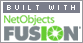Coming to Statistica
in 2022/2023

Zoom tutorials
using Explaineverything app
We a record sessions for you to listen to later

CALL STATISTICA NOW

.

E-mail     anne@statistica.com.au

# Place         Willetton, Western AustraliaTutorial  Topic One            Basic Number Rules (-3 - 7) and (-4) x (-5),    BIDMAS 23 +7/3 -(4 -2 - 8),                                                     Fractions a/b + c/d adding and a/b / c/d dividing Tutorial Topic Two               Solving and rearranging equations 4x +5 =20,  (s+1)/(s3-2) = (s+4)/(s4-12)                                                 Multiplying Brackets out (4c2 ( (5c+7) (c+1) ) )                   Solving Inequalities 3x +8 > 21 and  absolute values 3│4x + 7 │≥ 99 Tutorial  Topic Four             Finding the equation of Straight lines,using two points, and point/intercept                                                  and gradient, perpendicular  m1 m2 = - 1 and  parallel lines m1 = m2                                                 Linear Equations y = m x +c Simultaneous Equations 3y +4 =15 and 2y- x=5                                                  solve for x  and y  Tutorial Topic Five               Indices, powers (m2 n-2 /z n-4 m7 )4, solving square root problems ie Ö126,                                                  rationalising ‘opposite sign one’ Tutorial Topic Five extra     Cancelling Indices in detail Tutorial Topic Six                 Factorising quadratics, ax2 + bx + c, common factors for two or  four terms                                                  3x3 +9x, and  40ab -2b + 20ac  - cTutorial Topic Seven            Cancelling (s+2)/(s2-6s -16) and solving (adding /subtracting) algebraic                                                 fractions with  different factorising techniques                                                  (s+1)/(s2-6s -16) + (s+4)/(s2-16) = 1/ s2 ,                                                 Difference of two squares a2  – b2                                                                         Sum / difference of two cubed numbers  a3  ±   b3 Tutorial Topic Nine              Completing the square to find roots  (x  ± p )2 + q = 0,                                                  Quadratic formula to find roots    - b ±  Ö b2- 4 a c   = 0                                                                                                                            2 a                                                  Root form of quadratic   (s+1) (s - 6),  roots are s= -1 or s = 6                                                 Turning point form of quadratic (x  - p )2 + q, turning point co-ords are (p,q),                                                 Y intercept form of quadratic ax2 + bx + c,  crosses y axis at c Tutorial Topic Ten                Solving logarithms, log8 64   and powers 3 x = 81   Log Rules Tutorial Topic Ten Extra    Questions with growth/decay of form N(t) =N(0)e kt Tutorial Topic Eleven           Solving equations with logarithms 2 log x  +4 log y into one log                                                 and  powers  3x + 3x+1   = 81 Tutorial Topic Twelve          Basic Differentiation  3x ,  x-1/2   3Öx4  and finding the equation of a tangent                                                   line to a curveTutorial Topic Thirteen       Integration Basic  x (power+1) / (power +1)                                                 and Substitution where u = then ∫ u’s du Tutorial Topic  Fourteen      Area enclosed by curve and x-axis ,  Area between two curves Tutorial Topic Fifteen          Trigonometric equations Sin x = 0.5,  2 Sin 3x = 1 and equations  e(2y-7) =7[Home] [Parents] [Contact us] [Services] [Mathematics] [Statistics] [School]